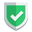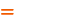我们相信：世界是美好的，你是我也是。平行空间的世界里面，不同版本的生活也在继续...

## 函数原型

``numpy.zeros(shape, dtype=None, order='C')``
``numpy.ones(shape, dtype=None, order='C')``
• `shape`是一个整数或整数元组，用于指定输出数组的形状。
• `dtype`是数据类型，默认为`float`
• `order`指定数组的存储顺序，默认为`C`，即按行存储。修改成`F`，意思是按列存储。

## 定义形状shape【最常用】

``````import numpy as np

s0 = np.array([0, 0, 0])
s1 = np.zeros(3)
s2 = np.ones((3,))
s3 = np.zeros((1, 3))
s4 = np.ones((3, 1))

print(s0, type(s0))
print(s1, type(s1))
print(s2, type(s2))
print(s3, type(s3))
print(s4, type(s4))``````

``````[0 0 0] <class 'numpy.ndarray'>
[0. 0. 0.] <class 'numpy.ndarray'>
[1. 1. 1.] <class 'numpy.ndarray'>
[[0. 0. 0.]] <class 'numpy.ndarray'>
[[1.]
[1.]
[1.]] <class 'numpy.ndarray'>``````

## 定义类型dtype【默认是float64】

``````import numpy as np

s0 = np.array([0, 0, 0])
s1 = np.zeros(3, dtype="int")
s2 = np.ones((3,), dtype="int32")
s3 = np.zeros((1, 3), dtype="float")
s4 = np.ones((3, 1), dtype="float64")
s5 = np.zeros((2, 2), dtype=int)
s6 = np.ones((2, 2), dtype=float)

print(s0, s0.dtype)
print(s1, s1.dtype)
print(s2, s2.dtype)
print(s3, s3.dtype)
print(s4, s4.dtype)
print(s5, s5.dtype)
print(s6, s6.dtype)``````

``````[0 0 0] int32
[0 0 0] int32
[1 1 1] int32
[[0. 0. 0.]] float64
[[1.]
[1.]
[1.]] float64
[[0 0]
[0 0]] int32
[[1. 1.]
[1. 1.]] float64``````

## 定义存储顺序order【无感】

``````import numpy as np

s1 = np.zeros(3, dtype="int", order="C")
s2 = np.ones((3,), dtype="int32", order="F")

print(s1)
print(s2)``````

``````[0 0 0]
[1 1 1]``````

## 结束语如果本文对您有帮助，或者节约了您的时间，欢迎打赏瓶饮料，建立下友谊关系。本博客不欢迎：各种镜像采集行为。请尊重原创文章内容，转载请保留作者链接。【福利】 腾讯云最新爆款活动！1核2G云服务器首年50元！【源码】本文代码片段及相关软件，请点此获取更多信息【绝密】秘籍文章入口，仅传授于有缘之人python